您可以捐助，支持我们的公益事业。 1元 10元 50元 认证码：必填求知 文章 文库 Lib 视频 iProcess 课程 认证 咨询 工具 讲座 Modeler Code要资料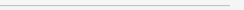订阅捐助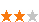396 次浏览     评价： 好 中 差
2019-8-27

 编辑推荐: 本文来自于csdn，文章主要通过举例说明挣值分析原理，CV和SV这两个值的典型偏差与非典型偏差，希望对您的学习有所帮助。

PV（计划值）在规定的时间内在工作上将要花费的获得批准的成本估算部分

AC（实际成本）在规定时间内完成工作所花费的实际成本（直接和间接成本的总额）

EV（挣值）实际完成工作的价值，完成了多少预算工作完成了多少预算工作.mypm.net

BAC （基线预算成本）全部工作的预算是多少？不改变成本基准，BAC就不会发生变化

CV（成本偏差）= EV-AC 费用偏差CV>0代表成本节约，好

SV（进度偏差）= EV-PV 进度偏差SV>0代表进度提前，好项目经理博

CV和SV这两个值，可以转化为效率指示器，反映任何工作项的成本与进度 计划绩效。

CPI（成本绩效指数）= EV/AC 费用指数CPI>1代表工作价值高，好

SPI（进度绩效指数）= EV/PV 效率指数SPI>1代表实际进度快，好项目管理论坛

CPI被广泛用于预测完工时的项目成本。SPI有时与CPI一起被用于预测项目完工估算。

ETC （完工时尚需成本估算]）：到完成时，剩余工作量还需要多少成本,ETC也就是估计完成项目的剩余工作成本

EAC （完成预估） ：全部工作的成本是多少？是根据项目的绩效和风险量化对项目最可能的总成本所做的一种预测典型偏差：

EAC= AC+(BAC-EV) =BAC/CPI

ETC=EAC-AC

ETC=BAC-EV基线总成本-已挣得部份

EAC=ETC+AC举例

PV=30+70+60*2/8+135+0+0=250(万元)

AC=35.5+83+17.5+159+0+0=295(万元)

EV=30*100%+70*100%+60*20%+135*100%+30*0%+70*0%=247(万元)

SV=EV-PV=247-250=-3

CV=EV-AC=247-295=-48

SPI=EV/PV=247/250=0.988

CPI=EV/AC=247/295=0.837

【问题2】

BAC=30+70+60*3/8+135+30*1/3=267.5万元

EAC=BAC/CPI=267.5*295/247=319.48万元

ETC=EAC-AC=319.48-295=24.48万元

ETC=BAC-EV=267.5-247=20.5万元

EAC=ETC+AC=20.5+295=315.5万元

【问题3】

1、建立成本基准计划（即确定计划值PV）

【例子】 中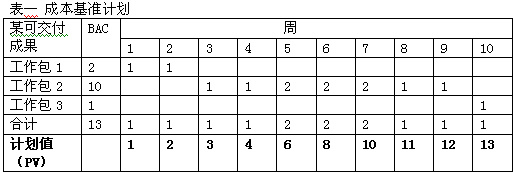2、记录和计算实际成本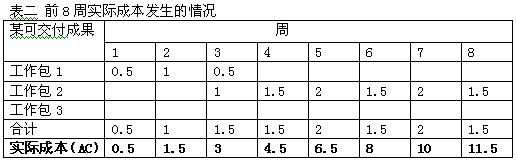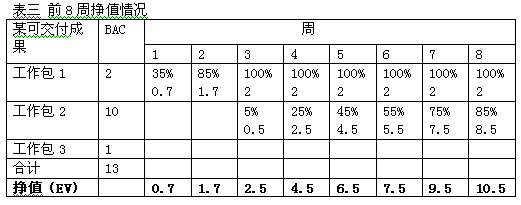396 次浏览  评价: 好 中 差订阅捐助
 相关文章 没人教的项目管理方法 软件项目管理流程总结 有效改进项目管理技能的十个过程 软件项目管理的流程控制分析
 相关文档 项目执行管理 软件项目开发过程 项目管理框架精华 软件开发项目管理
 相关课程 敏捷项目管理实践 软件项目管理 IT外包项目管理 基于功能点的工作量估算